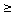# Averages, Arithmetic and Harmonic Means

Consider two similar problems (there is a more formal discussion):

### Problem 1

A fellow travels from city A to city B. For the first hour, he drove at the constant speed of 20 miles per hour. Then he (instantaneously) increased his speed and, for the next hour, kept it at 30 miles per hour. Find the average speed of the motion.

### Problem 2

A fellow travels from city A to city B. The first half of the way, he drove at the constant speed of 20 miles per hour. Then he (instantaneously) increased his speed and traveled the remaining distance at 30 miles per hour. Find the average speed of the motion.The two problems are often confused and the difference between them may not be immediately obvious. The second one, as a mathematical conundrum, has been included in many math puzzles books.

By definition, the average speed S of the motion that lasted time T over the distance D is

Average speed = (Total distance)/(Total time) or S = D/T

The definition applies directly to the first problem. The fellow was on the road for the total of T = 2 hours. Going at 20 mi/h for the first hour the fellow covered the distance of 20 miles. Similarly, in the second hour he covered the distance of 30 miles. Therefore, in 2 hours he traveled the total of D = 20 + 30 = 50 miles. The average speed then is found to be S = 50/2 = 25 mph.

The second problem is only a little more complex. There are three ways to write the formula above: S = D/T, D = ST, T = D/S. I apply the latter one. Let d be half the distance between the two cities. The first leg of the journey took d/20 hours, the second d/30. Therefore, on the whole, the fellow was on the road T = d/20 + d/30 hours. During that time he covered D = 2d miles. It follows that his average speed is given by S = 2d/(d/20 + d/30) or, after cancelling out the common factor d, S = 2/(1/20 + 1/30) = 24 mph. If the distance between the cities were, as in the first problem, 50 miles, then the journey would take T = 50/24 hours or 2 hours and 5 minutes, from which 1 hour and 15 minutes (= 25/20 hours) were spent on the first 25 miles and 50 minutes (= 25/30 hours) on the second 25 mile stretch.

What we found is that, depending on the circumstances, to determine the average speed of motion, computations based on the same basic formula (S = D/T) may have to follow different routes.There is another way to look at the situation. Let there be a relay race between two teams each consisting of two athletes. In one of the teams, the speed of the first athlete is 20 mph, that of the second is 30 mph. In the second team, two identical twins always run at the same speed. The question is, What must be the speed of the runners in the second team so that the two teams finish at exactly same time? The answer, as before, is 24 mph.

Watch the teams compete.

### If you are reading this, your browser is not set to run Java applets. Try IE11 or Safari and declare the site https://www.cut-the-knot.org as trusted in the Java setup.What if applet does not run?

For the given two quantities, 20 and 30, the number (20 + 30)/2 is known as their arithmetic mean while 2/(1/20 + 1/30) is the harmonic mean of the two numbers. We just observed that the arithmetic mean (the average) of two numbers 20 and 30 is not less than their harmonic mean. This is in fact true for any two numbers. Moreover, it is possible to define the arithmetic and harmonic means for any finite set of numbers and prove that the arithmetic mean is usually the larger of the two. They may also be equal. This only happens when all numbers in the set are equal.

For example, for 5 numbers a1, a2, a3, a4, and a5, the arithmetic mean - the avreage - is defined as

A = (a1 + a2 + a3 + a4 + a5)/5

whilst the harmonic mean is defined as

H = 5/(1/a1 + 1/a2 + 1/a3 + 1/a4 + 1/a5)

It can be shown that always AH with equality only when all numbers are equal.• The Means
• Expectation
• The Size of a Class: Two Viewpoints
• Averages of divisors of a given integer
• Family Statistics: an Interactive Gadget
• Averages in a sequence
• Arithmetic and Geometric Means
• Geometric Meaning of the Geometric Mean
• A Mathematical Rabbit out of an Algebraic Hat
• AM-GM Inequality
• The Mean Property of the Mean
• Harmonic Mean in Geometry
•